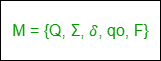# Difference between DFA and NFA

1. DFA: DFA refers to Deterministic Finite Automaton. A Finite Automata(FA) is said to be deterministic if corresponding to an input symbol, there is a single resultant state i.e. there is only one transition. A deterministic finite automata is set of five tuples represented as,Where,
Q: A non-empty finite set of states in the finite control(qo, q1, q2, …).
Σ: A non-empty finite set of input symbols.
δ: It is a transition function that takes two arguments, a state, and an input symbol, it returns a single state.
qo: It is starting state, one of the states in Q.
F: It is a non-empty set of final states/ accepting states from the set belonging to Q.

2. NFA:
NFA refers to Nondeterministic Finite Automaton. A Finite Automata(FA) is said to be non-deterministic if there is more than one possible transition from one state on the same input symbol.
A non-deterministic finite automata is also a set of five tuples and represented as,Where,
Q: A set of non empty finite states.
Σ: A set of non empty finite input symbols.
δ: It is a transition function that takes a state from Q and an input symbol from and returns a subset of Q.
qo: Initial state of NFA and member of Q.
F: A non-empty set of final states and member of Q.

Prerequisite – Finite Automata

### Difference between DFA and NFA :

DFA

NFA

DFA stands for Deterministic Finite Automata. NFA stands for Nondeterministic Finite Automata.
For each symbolic representation of the alphabet, there is only one state transition in DFA. No need to specify how does the NFA react according to some symbol.
DFA cannot use Empty String transition. NFA can use Empty String transition.
DFA can be understood as one machine. NFA can be understood as multiple little machines computing at the same time.
In DFA, the next possible state is distinctly set. In NFA, each pair of state and input symbol can have many possible next states.
DFA is more difficult to construct. NFA is easier to construct.
DFA rejects the string in case it terminates in a state that is different from the accepting state. NFA rejects the string in the event of all branches dying or refusing the string.
Time needed for executing an input string is less. Time needed for executing an input string is more.
All DFA are NFA. Not all NFA are DFA.
DFA requires more space. NFA requires less space then DFA.

eg: if we give input as 0 on q0 state so we must give 1 as input to q0 as self loop.